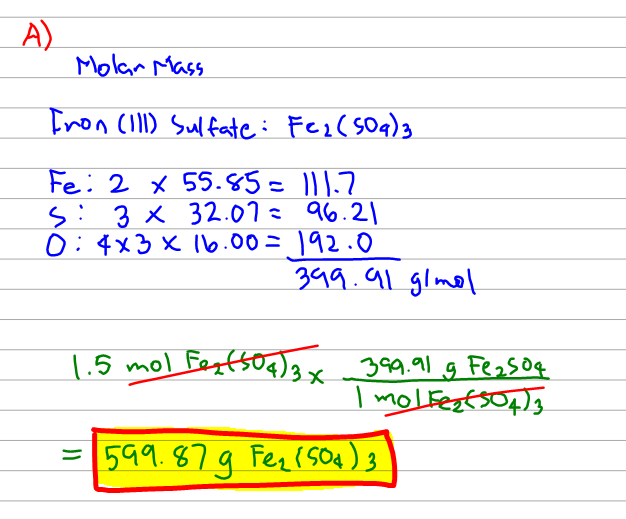# Problem: A) What is the mass, in grams, of 1.50 mol of iron (III) sulfate? Express your answer using three significant figures.B) How many moles of ammonium ions are in 6.935 g of ammonium carbonate? Express your answer using three significant figures.C) What is the mass, in grams, of 1.40 x 1021 molecules of aspirin, C9H8O4? Express your answer using three significant figures.

###### FREE Expert Solution###### Problem Details

A) What is the mass, in grams, of 1.50 mol of iron (III) sulfate? Express your answer using three significant figures.

B) How many moles of ammonium ions are in 6.935 g of ammonium carbonate? Express your answer using three significant figures.

C) What is the mass, in grams, of 1.40 x 1021 molecules of aspirin, C9H8O4? Express your answer using three significant figures.

What scientific concept do you need to know in order to solve this problem?

Our tutors have indicated that to solve this problem you will need to apply the Mole Concept concept. You can view video lessons to learn Mole Concept. Or if you need more Mole Concept practice, you can also practice Mole Concept practice problems.

What is the difficulty of this problem?

Our tutors rated the difficulty ofA) What is the mass, in grams, of 1.50 mol of iron (III) sul...as medium difficulty.

How long does this problem take to solve?

Our expert Chemistry tutor, Dasha took 10 minutes and 27 seconds to solve this problem. You can follow their steps in the video explanation above.

What professor is this problem relevant for?

Based on our data, we think this problem is relevant for Professor Briggs' class at ASU.